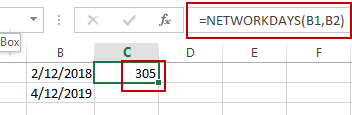## How to Calculate Number of Weekends between Two Dates in Excel

This post will guide you how to count the number of weekends between two given dates in Excel. How to find out how many weekends are between two dates in Excel. How to count the number of Sundays between two dates with a formula in Excel.

## Calculate the Number of Weekdays between Two Dates

Assuming that you have a list of data in range A1:B4, in which contain start dates and end dates. And you want to calculate the number of weekend days between start date and end date. How to do it. You can use a formula based on the NETWORKDAYS function and the DAYs function to calculate the number of weekends between two given dates.

Here is the formula that it will count the total number of weekend days between two given dates:

`=DAYS(B2,A2)+1-NETWORKDAYS(A2,B2)`

Type this formula into cell C2, and press Enter key on your keyboard, and then copy this formula from cell C2 to range C3:C4 to apply this formula.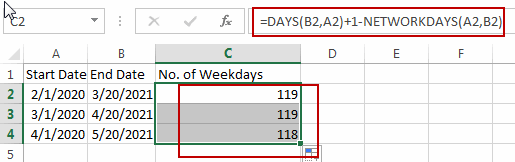Let’s see how this formula works:

The NETWORKDAYS function try to calculate the number of working days (weekdays) between two dates, and the DAYS function will count the number of days between two dates. So you can use days number to subtract working days, then you will get the number of weekends days.

## Calculate the Number of Sundays between Two Dates

If you only want to count the number of Sundays between two given dates in Excel, you can use an Excel formula based on the INT function and the WEEKDAY function. Like this:

`=INT((WEEKDAY(B1- 1)-A2+B2)/7)`

Type this formula into cell D2, and press Enter key on your keyboard.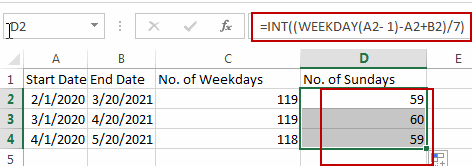Note: if you want to count the number of other week numbers (1-7, 1 is Sunday, 2 is Monday, 3 is Tuesday, 4 is Wednesday, 5 is Thursday, 6 is Friday, 7 is Saturday ) between two dates, such as: Saturday, you just need to replace the number 1 with 7 in the above formula. Like this:

`=INT((WEEKDAY(B1- 7)-A2+B2)/7)`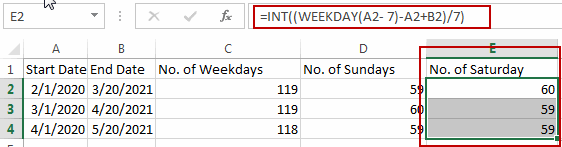### Related Functions

• Excel INT function
The Excel INT function returns the integer portion of a given number. And it will rounds a given number down to the nearest integer.The syntax of the INT function is as below:= INT (number)…
• Excel WEEKDAY function
The Excel WEEKDAY function returns a integer value representing the day fo the week for a given Excel date and the value is range from 1 to 7.The syntax of the WEEKDAY function is as below:=WEEKDAY (serial_number,[return_type])…
• Excel DAYS function
The Excel DAYS function returns the number of days between two dates.The syntax of the DAYS function is as below:= DAYS (end_date,start_date)…

## Count the Number of Weekends between Two Date

This post will guide you how to count the number of weekends between two dates in Excel. How do I get the number of weekends between 2 dates with a formula in Excel. How to count weekend days between dates in Excel.

## Count the Number of Weekends between Two dates

If you want to calculate the number of weekends between two dates, you need to create a formula based on the SUM function, the INT function and the WEEKDAY function. For example, you have two dates in Cell B2:C2, and the start date is in Cell B2, and the end date is in Cell C2. You can use the following formula to achieve the result.

`=SUM(INT((WEEKDAY(B2-{1,7})+C2-B2)/7))`

Type this formula into a blank cell and then press Enter key in your keyboard.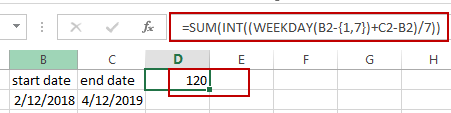The number of Weekends between those two dates is calculated.

Or you can use another formula to achieve the same result. Type the following formula into a blank cell and press Enter key.

`=C2-B2+1-NETWORKDAYS(B2,C2)`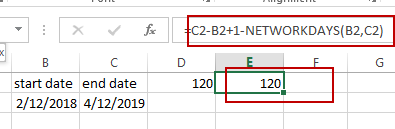### Related Functions

• Excel SUM function
The Excel SUM function will adds all numbers in a range of cells and returns the sum of these values. You can add individual values, cell references or ranges in excel.The syntax of the SUM function is as below:= SUM(number1,[number2],…)…
• Excel INT function
The Excel INT function returns the integer portion of a given number. And it will rounds a given number down to the nearest integer.The syntax of the INT function is as below:= INT (number)…
• Excel WEEKDAY function
The Excel WEEKDAY function returns a integer value representing the day fo the week for a given Excel date and the value is range from 1 to 7.The syntax of the WEEKDAY function is as below:=WEEKDAY (serial_number,[return_type])…

## Count the Number of Workdays between Two Dates

This post will guide you how to count the number of workdays between two dates in Excel. How do I get workdays between dates with a formula in Excel. How to calculate the number of working days between two dates in Excel.

## Count the Number of Workdays between Two Dates

If you want to calculate the number of workdays between two dates in Excel, you can use a formula based on the NETWORKDAYS function. And it will exclude all weekend days between the start dates and end dates. You need to specify two dates contain start date and end date so that you can get the number of workdays.

For example, you have two dates in range B1:B2, and you want to get the workdays between these two dates, you just need to use the following formula:

`=NETWORKDAYS(B1,B2)`

Type this formula in a blank Cell, and press Enter key in your keyboard.